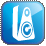# Speaker Tech Terms

By: |

Speaker Tech Answer Sheet

Score 2 points for every correct answer

 Multiple Choice Answers: 1.      A 2.      B 3.      A 4.      A 5.      B 6.      A 7.      B 8.      B 9.      B 10.  A 11.  A 12.  D 13.  B 14.  D 15.  D 16.  B 17.  B 18.  C 19.  B 20.  D 21.  D 22.  B 23.  C 24.  A 25.  D 26.  A 27.  D 28.  C 29.  B 30.  B True or False Answers A.     True B.     True C.     True D.     True E.     True F.      False G.    True H.    False I.       True J.      False K.    True L.     True M.   False N.     True O.    False P.      True Q.    False R.     True S.      True T.     True

Copy editor: Dan Rubin

This site uses Akismet to reduce spam. Learn how your comment data is processed.

Popups Powered By : XYZScripts.com